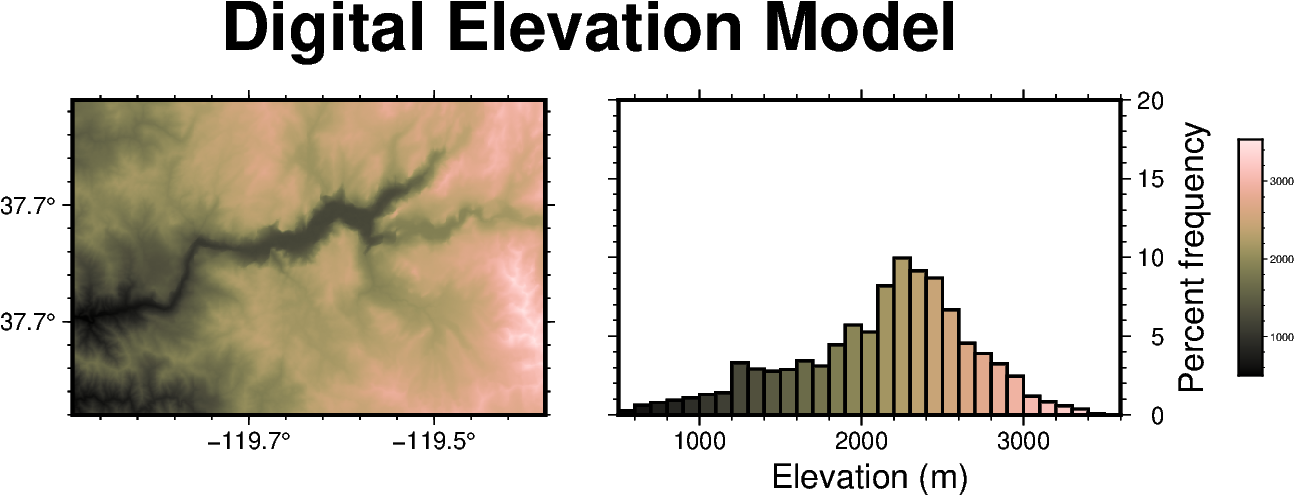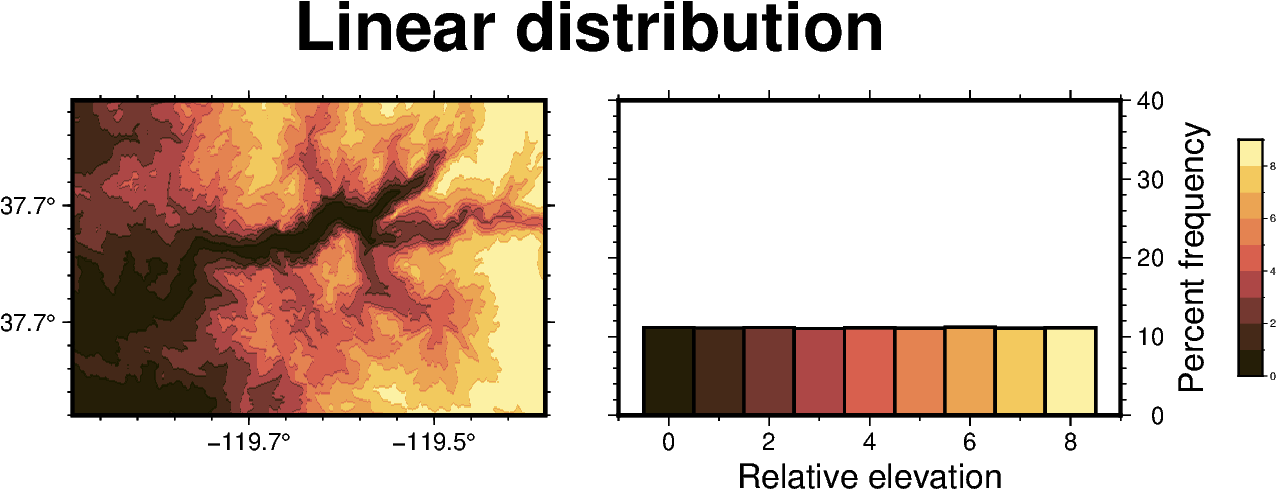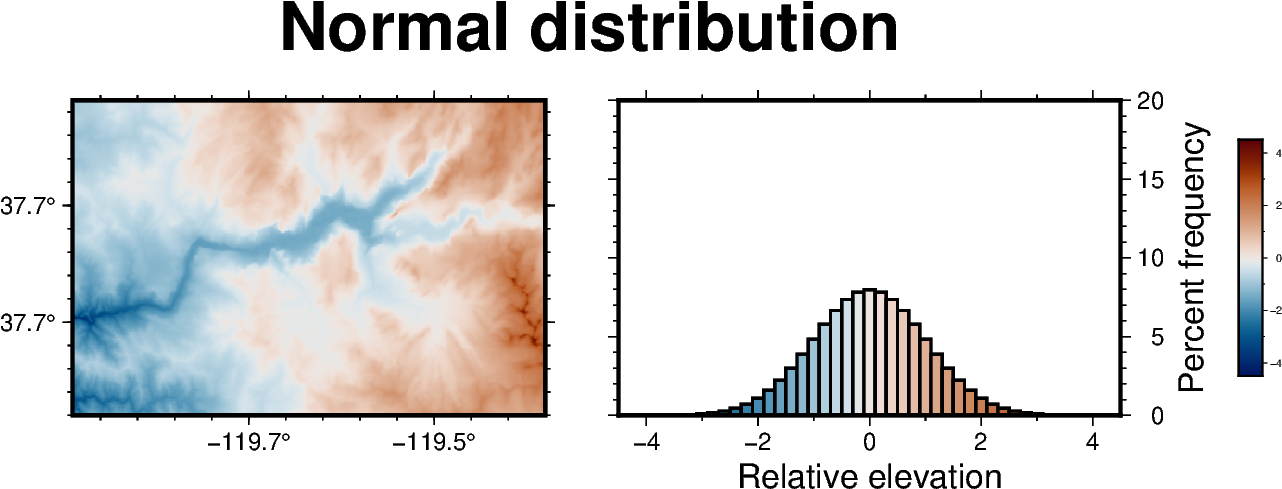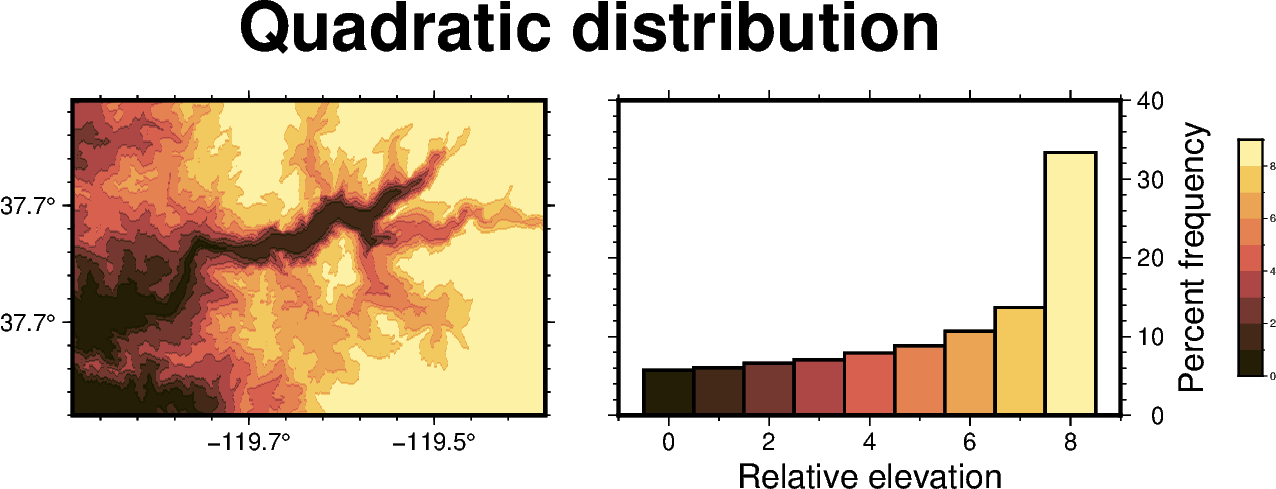# Performing grid histogram equalization

The `pygmt.grdhisteq.equalize_grid` method creates a grid using statistics based on a cumulative distribution function.

```import pygmt
```

Load the sample Earth relief data for a region around Yosemite valley and use `pygmt.grd2xyz` to create a `pandas.Series` with the z-values.

```grid = pygmt.datasets.load_earth_relief(
resolution="03s", region=[-119.825, -119.4, 37.6, 37.825]
)
grid_dist = pygmt.grd2xyz(grid=grid, output_type="pandas")["elevation"]
```

## Plot the original digital elevation model and data distribution

For comparison, we will create a map of the original digital elevation model and a histogram showing the distribution of elevation data values.

```# Create an instance of the Figure class
fig = pygmt.Figure()
# Define figure configuration
pygmt.config(FORMAT_GEO_MAP="ddd.x", MAP_FRAME_TYPE="plain")
# Define the colormap for the figure
pygmt.makecpt(series=[500, 3540], cmap="turku")
# Setup subplots with two panels
with fig.subplot(
nrows=1, ncols=2, figsize=("13.5c", "4c"), title="Digital Elevation Model"
):
# Plot the original digital elevation model in the first panel
with fig.set_panel(panel=0):
fig.grdimage(grid=grid, projection="M?", frame="WSne", cmap=True)
# Plot a histogram showing the z-value distribution in the original digital
# elevation model
with fig.set_panel(panel=1):
fig.histogram(
data=grid_dist,
projection="X?",
region=[500, 3600, 0, 20],
series=[500, 3600, 100],
frame=["wnSE", "xaf+lElevation (m)", "yaf+lPercent frequency"],
cmap=True,
histtype=1,
pen="1p,black",
)
fig.colorbar(position="JMR+o1.5c/0c+w3c/0.3c", frame=True)
fig.show()
```## Equalize grid based on a linear distribution

The `pygmt.grdhisteq.equalize_grid` method creates a new grid with the z-values representing the position of the original z-values in a given cumulative distribution. By default, it computes the position in a linear distribution. Here, we equalize the grid into nine divisions based on a linear distribution and produce a `pandas.Series` with the z-values for the new grid.

```divisions = 9
linear = pygmt.grdhisteq.equalize_grid(grid=grid, divisions=divisions)
linear_dist = pygmt.grd2xyz(grid=linear, output_type="pandas")["z"]
```

## Calculate the bins used for data transformation

The `pygmt.grdhisteq.compute_bins` method reports statistics about the grid equalization. Here, we report the bins that would linearly divide the original data into 9 divisions with equal area. In our new grid produced by `pygmt.grdhisteq.equalize_grid`, all the grid cells with values between `start` and `stop` of `bin_id=0` are assigned the value 0, all grid cells with values between `start` and `stop` of `bin_id=1` are assigned the value 1, and so on.

```pygmt.grdhisteq.compute_bins(grid=grid, divisions=divisions)
```
start stop
bin_id
0 508.0 1352.0
1 1352.0 1719.0
2 1719.0 1972.0
3 1972.0 2156.0
4 2156.0 2270.0
5 2270.0 2391.0
6 2391.0 2520.0
7 2520.0 2739.0
8 2739.0 3533.0

## Plot the equally distributed data

Here we create a map showing the grid that has been transformed to have a linear distribution with nine divisions and a histogram of the data values.

```# Create an instance of the Figure class
fig = pygmt.Figure()
# Define figure configuration
pygmt.config(FORMAT_GEO_MAP="ddd.x", MAP_FRAME_TYPE="plain")
# Define the colormap for the figure
pygmt.makecpt(series=[0, divisions, 1], cmap="lajolla")
# Setup subplots with two panels
with fig.subplot(
nrows=1, ncols=2, figsize=("13.5c", "4c"), title="Linear distribution"
):
# Plot the grid with a linear distribution in the first panel
with fig.set_panel(panel=0):
fig.grdimage(grid=linear, projection="M?", frame="WSne", cmap=True)
# Plot a histogram showing the linear z-value distribution
with fig.set_panel(panel=1):
fig.histogram(
data=linear_dist,
projection="X?",
region=[-1, divisions, 0, 40],
series=[0, divisions, 1],
frame=["wnSE", "xaf+lRelative elevation", "yaf+lPercent frequency"],
cmap=True,
histtype=1,
pen="1p,black",
center=True,
)
fig.colorbar(position="JMR+o1.5c/0c+w3c/0.3c", frame=True)
fig.show()
```## Transform grid based on a normal distribution

The `gaussian` parameter of `pygmt.grdhisteq.equalize_grid` can be used to transform the z-values relative to their position in a normal distribution rather than a linear distribution. In this case, the output data are continuous rather than discrete.

```normal = pygmt.grdhisteq.equalize_grid(grid=grid, gaussian=True)
normal_dist = pygmt.grd2xyz(grid=normal, output_type="pandas")["z"]
```

## Plot the normally distributed data

Here we create a map showing the grid that has been transformed to have a normal distribution and a histogram of the data values.

```# Create an instance of the Figure class
fig = pygmt.Figure()
# Define figure configuration
pygmt.config(FORMAT_GEO_MAP="ddd.x", MAP_FRAME_TYPE="plain")
# Define the colormap for the figure
pygmt.makecpt(series=[-4.5, 4.5], cmap="vik")
# Setup subplots with two panels
with fig.subplot(
nrows=1, ncols=2, figsize=("13.5c", "4c"), title="Normal distribution"
):
# Plot the grid with a normal distribution in the first panel
with fig.set_panel(panel=0):
fig.grdimage(grid=normal, projection="M?", frame="WSne", cmap=True)
# Plot a histogram showing the normal z-value distribution
with fig.set_panel(panel=1):
fig.histogram(
data=normal_dist,
projection="X?",
region=[-4.5, 4.5, 0, 20],
series=[-4.5, 4.5, 0.2],
frame=["wnSE", "xaf+lRelative elevation", "yaf+lPercent frequency"],
cmap=True,
histtype=1,
pen="1p,black",
)
fig.colorbar(position="JMR+o1.5c/0c+w3c/0.3c", frame=True)
fig.show()
```## Equalize grid based on a quadratic distribution

The `quadratic` parameter of `pygmt.grdhisteq.equalize_grid` can be used to transform the z-values relative to their position in a quadratic distribution rather than a linear distribution. Here, we equalize the grid into nine divisions based on a quadratic distribution and produce a `pandas.Series` with the z-values for the new grid.

```quadratic = pygmt.grdhisteq.equalize_grid(
)
```

## Calculate the bins used for data transformation

We can also use the `quadratic` parameter of `pygmt.grdhisteq.compute_bins` to report the bins used for dividing the grid into 9 divisions based on their position in a quadratic distribution.

```pygmt.grdhisteq.compute_bins(grid=grid, divisions=divisions, quadratic=True)
```
start stop
bin_id
0 508.0 1155.0
1 1155.0 1375.0
2 1375.0 1605.0
3 1605.0 1821.0
4 1821.0 1972.0
5 1972.0 2131.0
6 2131.0 2245.0
7 2245.0 2391.0
8 2391.0 3533.0

## Plot the quadratic distribution of data

Here we create a map showing the grid that has been transformed to have a quadratic distribution and a histogram of the data values.

```# Create an instance of the Figure class
fig = pygmt.Figure()
# Define figure configuration
pygmt.config(FORMAT_GEO_MAP="ddd.x", MAP_FRAME_TYPE="plain")
# Define the colormap for the figure
pygmt.makecpt(series=[0, divisions, 1], cmap="lajolla")
# Setup subplots with two panels
with fig.subplot(
nrows=1, ncols=2, figsize=("13.5c", "4c"), title="Quadratic distribution"
):
# Plot the grid with a quadratic distribution in the first panel
with fig.set_panel(panel=0):
# Plot a histogram showing the quadratic z-value distribution
with fig.set_panel(panel=1):
fig.histogram(
projection="X?",
region=[-1, divisions, 0, 40],
series=[0, divisions, 1],
frame=["wnSE", "xaf+lRelative elevation", "yaf+lPercent frequency"],
cmap=True,
histtype=1,
pen="1p,black",
center=True,
)
fig.colorbar(position="JMR+o1.5c/0c+w3c/0.3c", frame=True)
fig.show()
```Total running time of the script: ( 0 minutes 7.699 seconds)

Gallery generated by Sphinx-Gallery